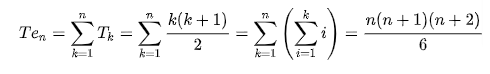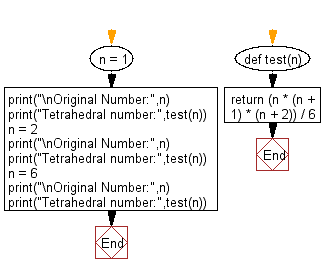﻿ Python Math: Get the nth tetrahedral number from a given integer(n) value - w3resource# Python Math: Get the nth tetrahedral number from a given integer(n) value

## Python Math: Exercise-84 with Solution

A tetrahedral number, or triangular pyramidal number, is a figurate number that represents a pyramid with a triangular base and three sides, called a tetrahedron. The formula for the nth tetrahedral number is represented by the 3rd rising factorial of n divided by the factorial of 3:Example of tetrahedral numbers:

N Tetrahedral
Number
11
24
310
420
535
656

Write a Python program to get the nth tetrahedral number from a given integer(n) value.

Sample Solution:

Python Code:

``````def test(n):
return (n * (n + 1) * (n + 2)) / 6

n = 1
print("\nOriginal Number:",n)
print("Tetrahedral number:",test(n))
n = 2
print("\nOriginal Number:",n)
print("Tetrahedral number:",test(n))
n = 6
print("\nOriginal Number:",n)
print("Tetrahedral number:",test(n))
```
```

Sample Output:

```Original Number: 1
Tetrahedral number: 1.0

Original Number: 2
Tetrahedral number: 4.0

Original Number: 6
Tetrahedral number: 56.0
```

Flowchart:## Visualize Python code execution:

The following tool visualize what the computer is doing step-by-step as it executes the said program:

Python Code Editor:

Have another way to solve this solution? Contribute your code (and comments) through Disqus.

What is the difficulty level of this exercise?

Test your Programming skills with w3resource's quiz.

﻿

```>>> students = [{'name': 'John', 'score': 98}, {'name': 'Mike', 'score': 94}, {'name': 'Jennifer', 'score': 99}]